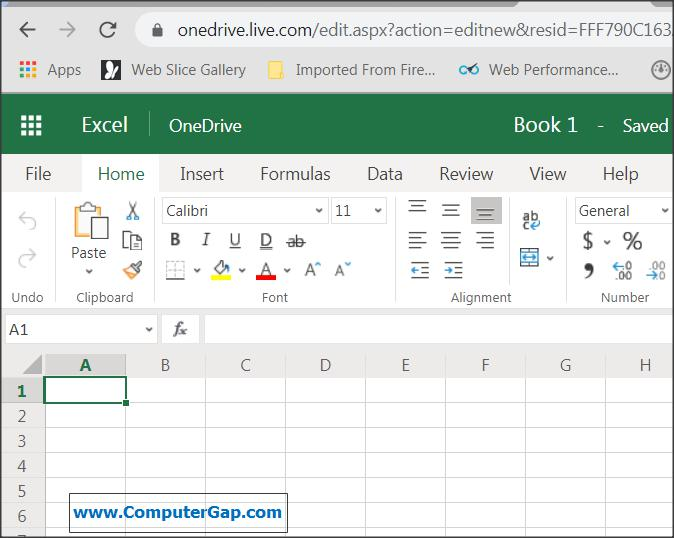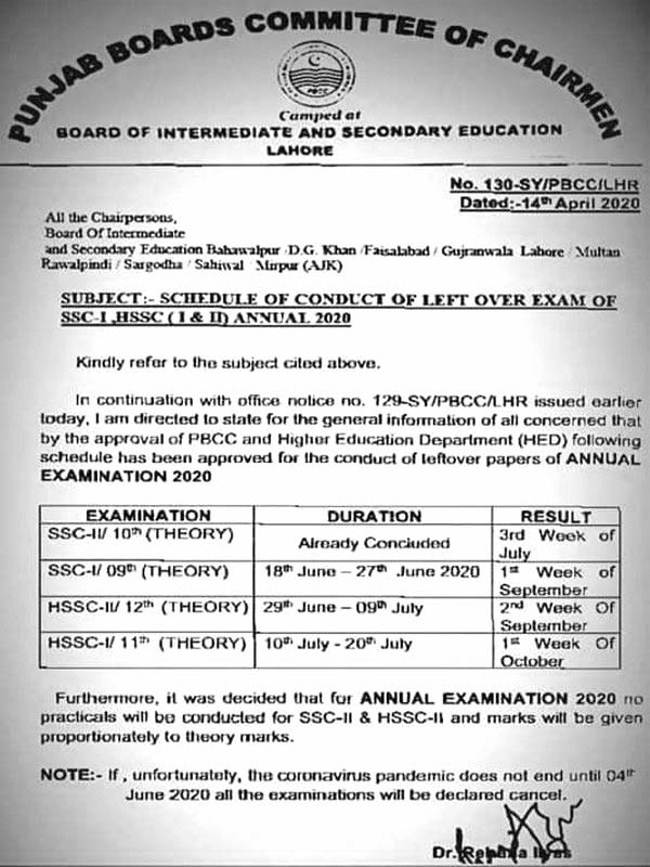# Perfect Computer Notes

Super Easy Short Computer Notes To Improve Computer Literacy and Your IT Skills

# Use Microsoft Excel Online [ 2020 ] Free Spreadsheet

Topic: Use Microsoft Excel Online Free Spreadsheet

### How To Create Excel Worksheet Online Free

Don't worry if you are to prepare a worksheet in Microsoft Excel and you have not purchased Microsoft Office software. You can use free online version of Microsoft Excel Online and create
worksheets as you like.Use Microsoft Excel Online Free Spreadsheet

Here are the easy steps to create  power calculator worksheet using Microsoft Excel Online free.

1. for this purpose you need to have a Microsoft Account.

https://office.live.com/start/excel.aspx

3. Login to your Microsoft account ( like abc@hotmail.com or xyz@hotmail.com) or read this post to Create a new Microsoft account in easy steps.

#### Select New Blank Workbook

4. Click on the New Blank Workbook option.

5. A blank Workbook will open. The worksheet named 'sheet1' is here to use for entring and processing your data.

6. Let's create a simple Power calculator worksheet.

7. Enter the sample data and apply some formatting as shown in the figure. Apply Merge and Center Option on the heading.

### Enter Sample Data in Excel Worksheet Online

8. similarly, you can apply font color and fill color options as shown in the figure while selecting the text.

### Insert a Function Power(number, power/exponent) in the required cell of the excel worksheet

9. This worksheet will use the function power(b3,c3) to calculate power of a number. The first number is in cell b3. the first power or exponent is in the cell C3.

10. After inserting the function in the cell D3, press Enter.

11. the result will be shown in the cell.

### Copy the Formula Downwards in Excel Sheet

12. Now copy the formula by taking the mouse pointer to bottom right corner of the cell containing the formula. When a + sign appears, click left mouse button and drag down for the cells to copy formula. Note that you must keep the left mouse button pressed as long as you drag down.

### Save Excel worksheet / Workbook Online

13. Now click on nine dot button from top left corner and save the excel workbook. This workbook will bbe saved inOneDrive online storage provided free by Microsoft.

14. You can rename the workbook or download your workbook in your own computer. For this purpose use the nine dot button and the options as shown in the figure.

# Matric and Intermediate Examination 2020 Schedule All Punjab Boards

Topic: Matric and Intermediate Examination 2020 Schedule All Punjab Boards

## میٹرک اور انٹرمیڈیٹ کے پریکٹیکل نہیں ہوں گے اور سٹوڈنٹس کے تھیوری میں حاصل کردہ نمبروں کے تناسب سے پریکٹیکل کے نمبر لگائے جائیں گےMatric and Intermediate Examination 2020 Schedule All Punjab Boards

# Introduction to Algorithms and Algorithmic Notation With Examples

Topic: Introduction to Algorithm and Algorithmic Notation With Examples

### What is an Algorithm?

The step by step procedure to solve a particular problem is called an Algorithm.  A Good Programmer always write algorithms before developing programs.Algorithmic Notation with example algorithms

### Advantages of  writing an Algorthm

The process of solving a problem becomes simpler and easier with the help of  algorithm.
There are less chance of errors in the program, if we design algorithms before writing our programs in any programming language.

### What is the Relationship between an Algorithm and a Program

An algorithm is the sequenc of steps to perform a task, whereas a program is the set of statements to perform a specific task. Normally, we will use a programming statement (instruction of a programming language) for each step of an algorithm.

For example, consider the following four steps of an algorithm of a program to find square of a number:
1. Print "Enter a number:"
2. Input a number in n.
3. Calculate square = n * n
4. Print "Square=",square
Now we will write the program instructions for the above steps of the algorithm:

```1. printf("Enter a number:");
2. scanf("%d",n);
3. square = n * n;
4. printf("Square=%d",square); ```

### Introduction to Algorithmic Notation

The algorithms are written using an algorithmic noataion. Normally there is no standard algorithmic otation. Every author uses his own set of algorithmic statements to design the algorithms.

For example, we will use the following set of statements as algorithmic notation to design the algorithms:

#### The Name of Algorithm

First of  all we will write the name of the algorithm for example:
Algorithm square.

#### The Description of Algorithm

Here we will describe the purpose of the algorithm.
For example:
// This algorithm is used to input a number and display its square.

The comments are used to explain the purpose of  a step of an algorithm. For example:
// Compute square of the number n
square = n * n
We will use double slash // to start a single line comment.

#### The Variables

A variable is an entity whose value can be changed. It is used to store data. We do not need to declare variables. We simply use variables in an algorithm according to the requirements. We will use simple variable names in small letters. For example, n or num1, num2, square, factorial, first_name etc.

### The Statements

There are differnt statements in an algorithmic notation to perform differnt tasks.

#### Input Statement

Inmput statement is used to input data into different variables.
For example:
Input a number in n.
Or more precisely
Input n
Note: rad is a variable to store the value of the radius of the circle.
Or more precisely,

#### Output statement

The output statement is used to display string messages and the values of variables:
For example:
Print "Enter a number"
or
Print "Sum=",sum

#### Assignment statement

Assignment statement is used to assign a value to a variable or to calculate a formula. For example,
i = 1
or
sum = num1 + num2

#### If statement

If statement is used to decide the execution of a statement or a set of statements if the given condition is true.
For example:
If (num1 == num2)
Print "both numbers are equal"
End If

#### If Else Statement

If else statement is a two way selection. If condition is true, the if block is executed otherwise, the else block of statements is executed:
If (num1 ==  num2)
Print "both numbers are equal"
Else
Print "numbers are different"
End If

#### Repeat - For

A Repeat - for construct is a looping statement. It is used to execute a block of one or more statements repeatedly for a fixed number of times. For example: the following for statement print 1 to 5 numbers.
Step 1. Repeat steps 1.1 For i = 1 to 5 step 1
Step 1.1 Print i
End For

#### Repeat - While

A Repeat - While statement is used to execute a block of one or more statements as long as the given condition remains true. For example: Input a number, and sum it as long as we enter a number gretare than zero.

``````
Step 1. sum = 0

Step 2. Print "Enter a number:"

Step 3. Input n

Step 3. Repeat Step 3.1,3.2,3.3 While ( n > 0)

Step 3.1  sum = sum + n

Step 3.2  Print "Enter a number:"

Step 3.3  Input n

End While

Step 4. Print "Sum=",sum

``````

## Algorithm to Add Two Numbers

Algorithm Sum
//This algorithm inputs two numbers and display their sum.
// It uses three integer variables num1, num2 and sum.
Step 1.  Start
Step 2.  Print "Enter two numbers to Add="
Step 3.  Input num1, num2
step  4.  Calculate sum = num1 + num2
Step 5.  Print "Sum=", sum
Step 6.  End

## Algorithm to Check whether Two Numbers are Equal

Algorithm Check_Equal
//This algorithm inputs two numbers and display if they are equal or not
// It uses two integer variables num1, num2
Step 1.  Start
Step 2.  Print "Enter two numbers to Add="
Step 3.  Input num1, num2
step  4.  If ( num1 == num2 )
Print "Both numbers are equal"
Else
Print "Both numbers are different"
End If
Step 5. End

### Algorithm To Find Factorial of a Number

Algorithm Factorial
//This algorithm inputs a number and displays its factorial using Repeat-While
Step 1. Start
Step 2. Print "Enter a number:"
Step 3. Input n
Step 4. Set fact = 1, i = 1
Step 5. Repeat Step 5.1 To 5.2 While (i<=n)
Step 5.1   Set fact = fact * i
Step 5.2   Set i = i + 1
End While
Step 6. Print "Factorial =", fact
Step 7. End

### Algorithms, Algorithmic Notation and Problem Solving

We have discussed the following concepts in this Algorithms and Algorithmic Notation tutorial:
Algorithms, Importance of Algorithm, Notation used to describe algorithms and some important examples of algorithms.